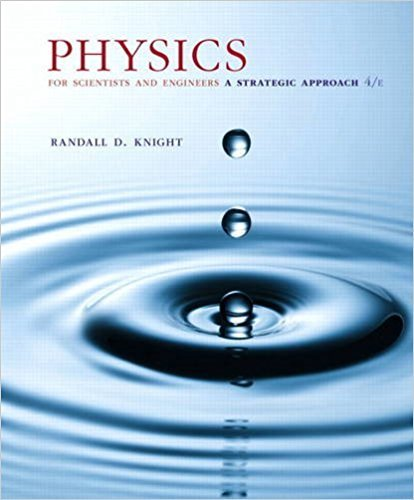×
×

# Prove that the expression for x1t2 in Equation 15.55 is aISBN: 9780134081496 191

## Solution for problem 15.108 Chapter 15

Physics for Scientists and Engineers: A Strategic Approach, Standard Edition (Chs 1-36) | 4th Edition

• Textbook Solutions
• 2901 Step-by-step solutions solved by professors and subject experts
• Get 24/7 help from StudySoup virtual teaching assistantsPhysics for Scientists and Engineers: A Strategic Approach, Standard Edition (Chs 1-36) | 4th Edition

4 5 0 268 Reviews
30
1
Problem 15.108

Prove that the expression for x1t2 in Equation 15.55 is a solution to the equation of motion for a damped oscillator, Equation 15.54, if and only if the angular frequency v is given by the expression in Equation 15.56

Step-by-Step Solution:
Step 1 of 3
Step 2 of 3

Step 3 of 3

##### ISBN: 9780134081496

Unlock Textbook Solution